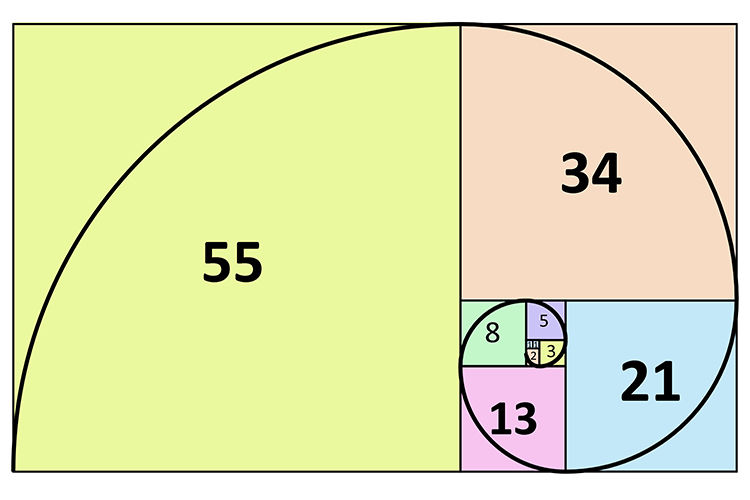top of page
Search

# Fibonacci Ratios

Updated: Sep 15, 2020

In the early 1200's, the Italian mathematician Leonardo Pisano Fibonacci uncovered a secret about this ancient civilization that would revolutionize the entire mathematical world... including the financial markets! While studying the Great Pyramid "Gizeh" in Egypt, Fibonacci made a startling discovery and uncovered a unique mathematical sequence of numbers that changed several theories of trigonometry, algebra & geometry. He developed the famous Fibonacci sequence of numbers, which has become the foundation of many effective trading systems.

Now these Fibonacci numbers which are used in art, music, biology and architecture, are also used in the financial markets to identify strong support/resistance levels.

The sequence, shown below, simply says that the third number is effectively the sum of previous two numbers - 1, 1, 2, 3, 5, 8, 13, 21, 34, 55, 89, 144, and so on.In this series, the real value lies in the fact, that the ratio of any number to the next higher number is approximately 0.618, and the lower number is 1.618.

The key Fibonacci ratio of 61.8% - also referred to as "the golden ratio" or "the golden mean" - is found by dividing one number in the series by the number that follows it. For example: 8/13 = 0.6153, and 55/89 = 0.6179.

The 38.2% ratio is found by dividing one number in the series by the number that is found two places to the right. For example: 55/144 = 0.3819.

The 23.6% ratio is found by dividing one number in the series by the number that is three places to the right. For example: 8/34 = 0.2352.It can accurately anticipate when the market makes a major turn & identify key turning points for tops and bottoms.

But, the truth is most traders seldom use it properly. And ironically, even though it is one of the most effective tools of technical analysis, it is also the most misunderstood. If you can learn to use them correctly, you can increase the probability of profitable trades & minimize potential losses.

Since Forex is a highly trending market, where prices are visibly changing in an oscillatory pattern, it follows the Fibonacci ratios quite precisely. These Fibonacci levels act as strong indicators of resistance and support levels, which helps to improve the accuracy of the entry and exit point for every trade. One of the immense advantages of trading with Fibonacci numbers is the fact that you can define not only stop losses to exit a market, but set profit objectives as well. The different Fibonacci ratios are the cornerstone of price structure and go hand-in-glove with effective chart patterns like Elliot waves, Harmonic patterns, Divergences etc.

The Fibonacci studies can be separated into four main groups:

1. Time Zones

2. Fans

3. Arcs

4. Retracements​For some reason, these ratios seem to play an important role in the financial markets, just as they do in nature, and can be used to determine critical points that cause price to reverse. Price has an uncanny way of respecting Fibonacci ratio’s, often quite precisely. Hence one can use the Fib ratios to ascertain the correct technical levels.

Price action is never random, and every wave leaves behind the clues for the next move. We can thus, use the previous price action to determine the anticipated price movement.

### ​

A common misinterpretation of the Fibonacci numbers is that traders tend to use the same Fibonacci ratio for all kinds of situations. The Fibonacci retracement tool is generally used as a standard tool for all kinds of situations, with the standard values of 38.2; 50.0; & 61.8.

But just like the different tools in a carpenter’s tool box, each ratio should be used in a particular situation.

### Using these ratios in a proper way gives us a tremendous advantage over the crowd & we have refined the different Fibonacci ratios to be used for specific technical patterns. These different Fibonacci ratios are the foundation of all our trading techniques such as the Harmonic patterns, Wolfe waves, Divergence etc.